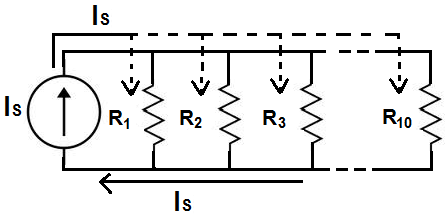﻿ Current Divider Calculator ﻿# Current Divider CalculatorEnter up to 10 resistors

 IS: A (amperes) mA (milliamperes) μA (microamperes) R1: Ω (ohms) KΩ (Kilohms) MΩ (Megohms) R2 Ω (ohms) KΩ (Kilohms) MΩ (Megohms) R3 Ω (ohms) KΩ (Kilohms) MΩ (Megohms) R4 Ω (ohms) KΩ (Kilohms) MΩ (Megohms) R5 Ω (ohms) KΩ (Kilohms) MΩ (Megohms) R6 Ω (ohms) KΩ (Kilohms) MΩ (Megohms) R7 Ω (ohms) KΩ (Kilohms) MΩ (Megohms) R8 Ω (ohms) KΩ (Kilohms) MΩ (Megohms) R9 Ω (ohms) KΩ (Kilohms) MΩ (Megohms) R10 Ω (ohms) KΩ (Kilohms) MΩ (Megohms)

This Current Divider Calculator can calculate the current going through any branch in a parallel circuit, using the formula shown above.

When a circuit is in parallel, the total current coming from the power source divides up into each of the branches of the circuit, based on the resistance values of each of the branches. Current takes the path of least resistance, so more current will go into branches with smaller resistance; conversely, less current will go into a branch of great resistance. For a tutorial on current division, visit Current Divider Circuit.

To calculate the current through any of the branches of the circuit, the formula is, IBRANCH = IS(RTOTAL/RBRANCH), where IBRANCH is the current through the individual branch, IS is the current from the current source, RTOTAL is the equivalent parallel resistance of all the resistors, and RBRANCH is the resistance of the individual branch.

To use this calculator, all a user must do is enter the value of the current coming from the current source and each of the resistances of the branches in the circuit. This is all the data needed to compute the current going through any of the various branches of a circuit.

You can enter in how ever many branches you need from 2 to 10 branches. Thus, if you have a circuit that only has 2 branches, you would just enter in values for the first 2 resistance values, and leave the rest of the fields blank. If you have a circuit that has 5 branches, then you would just enter in resistance values for the first 5 branches and leave the remaining 5 resistance fields blank. Technically, this calculator can calculate the current divider circuit with a single branch. However, this really isn't a current divider circuit. However, the calculator will simply give the output current of whatever current you enter (since the current doesn't divide up).

The unit of the result of this calculator is in the unit specified. The resultant value can either be in amperes (A), milliamperes (mA), or microamperes (μA).

Related Resources

﻿